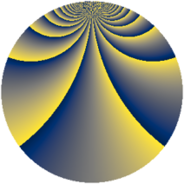# Properties

 Label 1148.2.clLevel $1148$ Weight $2$ Character orbit 1148.cl Rep. character $\chi_{1148}(11,\cdot)$ Character field $\Q(\zeta_{120})$ Dimension $5248$ Sturm bound $336$

# Learn more about

## Defining parameters

 Level: $$N$$ $$=$$ $$1148 = 2^{2} \cdot 7 \cdot 41$$ Weight: $$k$$ $$=$$ $$2$$ Character orbit: $$[\chi]$$ $$=$$ 1148.cl (of order $$120$$ and degree $$32$$) Character conductor: $$\operatorname{cond}(\chi)$$ $$=$$ $$1148$$ Character field: $$\Q(\zeta_{120})$$ Sturm bound: $$336$$

## Dimensions

The following table gives the dimensions of various subspaces of $$M_{2}(1148, [\chi])$$.

Total New Old
Modular forms 5504 5504 0
Cusp forms 5248 5248 0
Eisenstein series 256 256 0

## Trace form

 $$5248q - 16q^{2} - 20q^{4} - 32q^{5} - 64q^{6} - 64q^{8} - 32q^{9} + O(q^{10})$$ $$5248q - 16q^{2} - 20q^{4} - 32q^{5} - 64q^{6} - 64q^{8} - 32q^{9} - 12q^{10} - 40q^{12} - 128q^{13} - 44q^{14} - 12q^{16} - 32q^{17} - 12q^{18} - 80q^{20} - 64q^{21} - 16q^{22} + 8q^{24} - 40q^{25} - 16q^{26} - 48q^{28} - 128q^{29} + 8q^{30} - 36q^{32} - 32q^{33} - 64q^{34} - 32q^{36} - 24q^{37} - 4q^{38} - 128q^{41} - 64q^{42} - 48q^{44} - 40q^{45} - 72q^{46} - 200q^{48} - 32q^{49} - 88q^{50} + 72q^{52} - 32q^{53} + 64q^{54} - 48q^{56} - 96q^{57} + 12q^{58} - 40q^{60} - 32q^{61} - 80q^{62} - 80q^{64} - 48q^{65} - 20q^{66} + 52q^{68} - 176q^{69} + 4q^{70} - 20q^{72} - 32q^{73} + 12q^{74} - 384q^{76} - 64q^{77} + 160q^{78} - 44q^{80} + 176q^{82} - 200q^{84} - 64q^{85} - 20q^{86} - 60q^{88} - 32q^{89} - 640q^{90} - 224q^{92} - 80q^{93} + 116q^{94} + 200q^{96} - 128q^{97} + 28q^{98} + O(q^{100})$$

## Decomposition of $$S_{2}^{\mathrm{new}}(1148, [\chi])$$ into newform subspaces

The newforms in this space have not yet been added to the LMFDB.